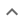•# Factoring multivariable polynomials

We think you wrote:

###This solution deals with factoring multivariable polynomials.

Solution found(x+2y)*(2x+9y)

## Step by Step Solution## Step  1  :

#### Equation at the end of step  1  :

  ((2 • (x2)) +  13xy) +  (2•32y2)
Step  2  :

#### Equation at the end of step  2  :

  (2x2 +  13xy) +  (2•32y2)


## Step  3  :

#### Trying to factor a multi variable polynomial :

Factoring    2x2 + 13xy + 18y2

Try to factor this multi-variable trinomial using trial and error

Found a factorization  :  (x + 2y)•(2x + 9y)

## Final result :

  (x + 2y) • (2x + 9y)